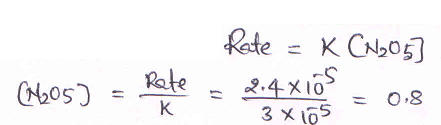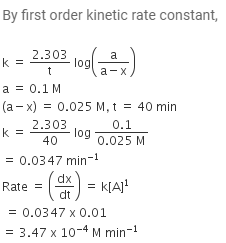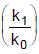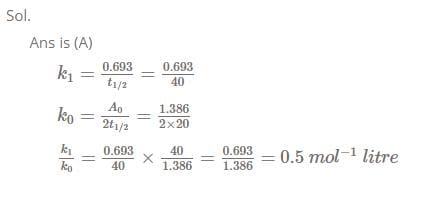# MCQ (Previous Year Questions) - Chemical Kinetics (Level 2)

## 11 Questions MCQ Test Chemistry for JEE Advanced | MCQ (Previous Year Questions) - Chemical Kinetics (Level 2)

Description
Attempt MCQ (Previous Year Questions) - Chemical Kinetics (Level 2) | 11 questions in 22 minutes | Mock test for JEE preparation | Free important questions MCQ to study Chemistry for JEE Advanced for JEE Exam | Download free PDF with solutions
QUESTION: 1

### Plots showing the variation of the rate constant (k) with temperature (T) are given below. The plot that follows Arrhenius equation is             [JEE 2010]

Solution:

Option (A) is correct. K increases exponentially with a rise in T(K=Ae−Ea​/RT).

The Arrhenius plot is used to study the effect of temperature on reaction rates. The Arrhenius plot can also be used by extrapolating the line back to the y-intercept to obtain the pre-exponential factor, A.

This factor is significant because A=pZ, where p is a steric factor and Z is the collision frequency. The pre-exponential, or frequency, the factor is related to the number of times molecules will hit in the orientation necessary to cause a reaction.

QUESTION: 2

### The rate constant for the reaction 2N2O5 → 4NO2 + O2 is 3.0 × 10–5 sec–1. If the rate is 2.4 × 10–5 mol litre–1 sec–1, then the concentration of N2O5 (in mol litre–1) is           [JEE SCR 2000]

Solution:QUESTION: 3

### If I is the intensity of absorbed light and C is the concentration of AB for the photochemical process AB + hv → AB*, the rate of formation of AB* is directly proportional to           [JEE SCR 2001]

Solution:
In photo initiated primary process rate of reaction is directly proportional to intensity of light uses.
Hence B is the correct answer.
QUESTION: 4

Consider the chemical reaction, N2(g) + 3H2(g) → 2NH3(g). The rate of this reaction can be expressed in term of time derivative of concentration of N2(g), H2(g) or NH3(g). Identify the correct relationship amongst the rate expressions.           [JEE SCR 2002]

Solution:
QUESTION: 5

In a first order reaction the concentration of reactant decreases from 800 mol/dm3 to 50 mol/dm3 in 2
× 104 sec. The rate constant of reaction in sec–1 is :           [JEE SCR 2003]

Solution:
QUESTION: 6

The reaction, X → Product follows first order kinetics. In 40 minutes the concentration of X changes from 0.1 M to 0.025 M. Then the rate of reaction when concentration of X is 0.01 M           [JEE SCR 2004]

Solution:QUESTION: 7

Which of the following statement is incorrect about order of reaction ?           [JEE 2005]

Solution:
QUESTION: 8

Consider a reaction aG + bH → Products. When concentration of both the reactants G and H is doubled, the rate increases by eight times. However, when concentration of G is doubled keeping the concentration of H fixed, the rate is doubled. The overall order of the reaction is           [JEE 2006]

Solution: AG + bH > product

G H
2x 2x > 8r
2x x >2r

Therefore,
r =[H]^2 [G]
Hence,
order =2+1=3
Correct option,
(D)
QUESTION: 9

Under the same reaction conditions, initial concentration of 1.386 mol dm–3 of a substance becomes
half in 40 seconds and 20 seconds through first order and zero order kinetics, respectively.

Ratioof the rate constants for first order (k1) and zero order (k0) of the reactions is [JEE 2008]

Solution:QUESTION: 10

For a first order reaction A → P, the temperature (T) dependent rate constant (k) was found to follow the equation log k = – (2000) 1/T + 6.0. The pre-exponential factor A and the activation energy Ea, respectively, are           [JEE 2009]

Solution:
*Multiple options can be correct
QUESTION: 11

For the first order reaction
2N2O5(g) → 4NO2(g) + O2(g)           [JEE 2011]

Solution:Use Code STAYHOME200 and get INR 200 additional OFF Use Coupon Code